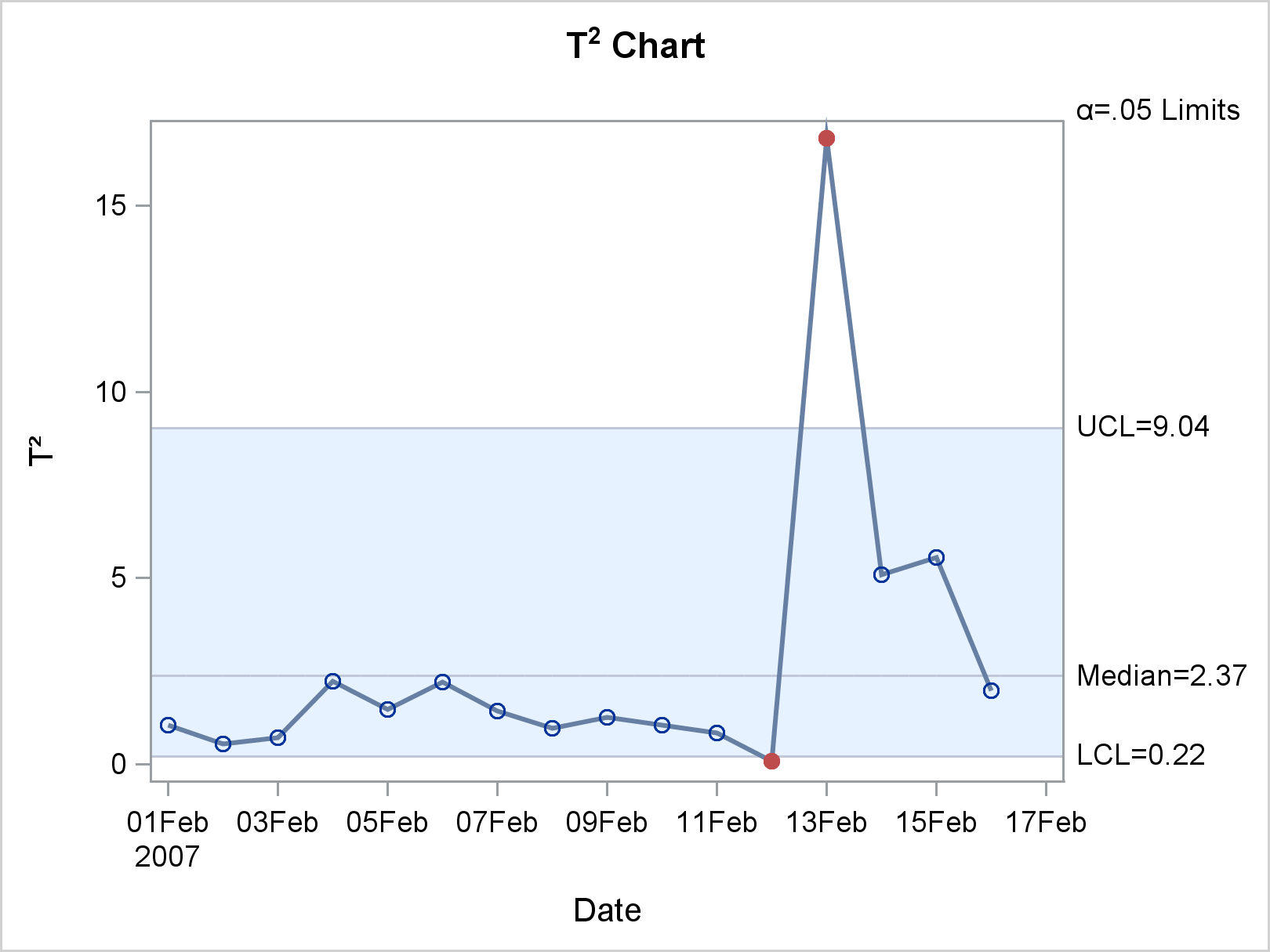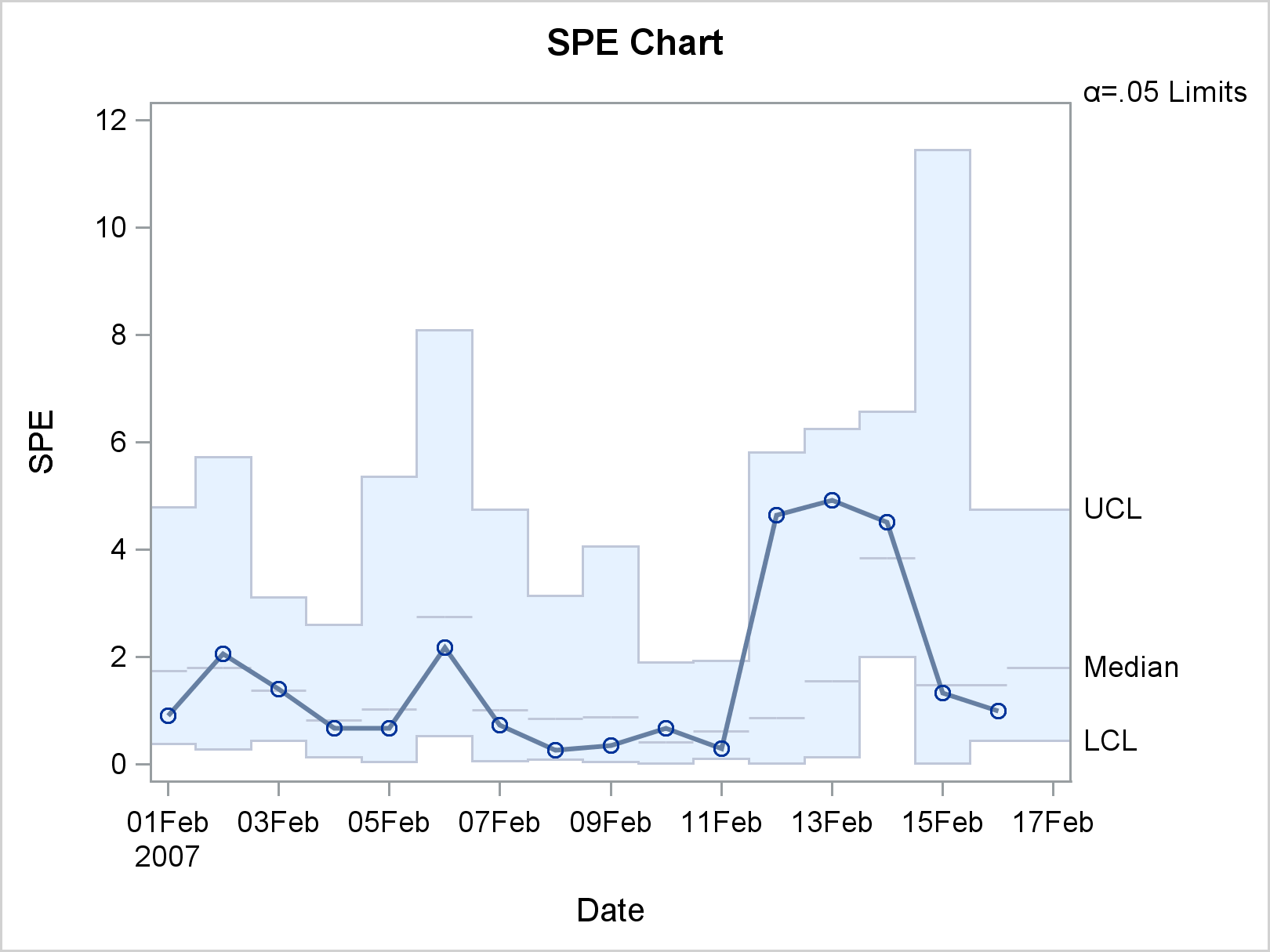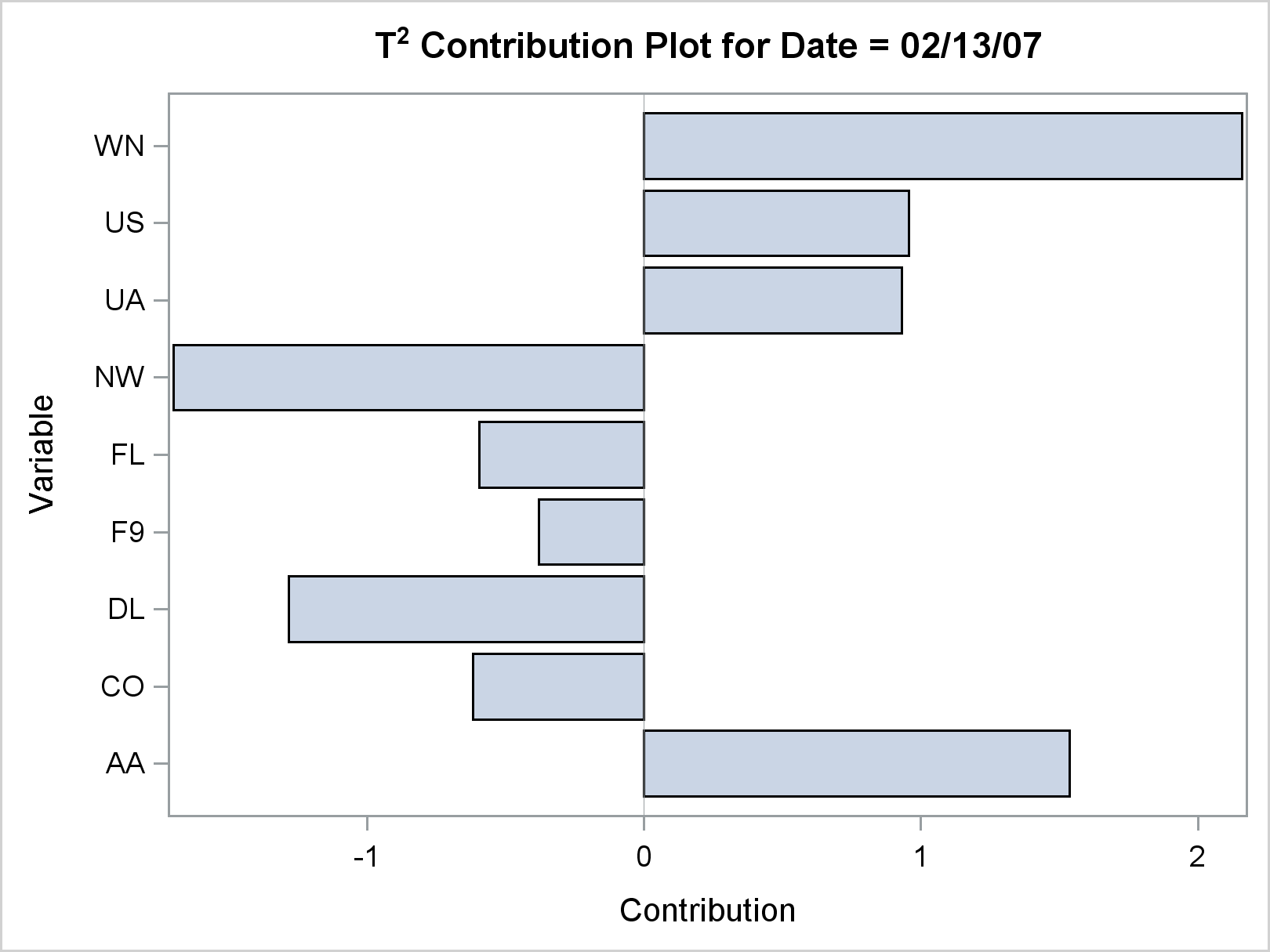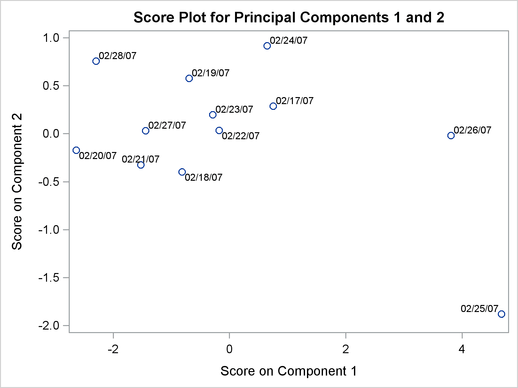SAS/QC Software

Multivariate Process Monitoring

The MVPMODEL and MVPMONITOR procedures are used together to monitor multivariate process variation over time in order to determine whether a process is stable or to detect and diagnose changes in a stable process. The MVPMODEL procedure builds statistical models, which the MVPMONITOR procedure then uses to create multivariate control charts.

Multivariate control charts detect unusual variation that would not necessarily be discovered by individually monitoring the measured variables with univariate control charts such as Shewhart charts. A multivariate control chart detects changes in the linear relationships of the variables in addition to changes in their marginal means and variances.

The MVPMODEL procedure constructs principal components analysis (PCA) models, which represent the data with a small number of principal components that capture most of the variation. The model-building process provides cross validation plots, which are useful for determining the number of principal components, in addition to plots of the principal component loadings and scores, scree plots, and plots of the variance proportion explained.

The MVPMONITOR procedure produces control charts for multivariate process data. It reads data sets that contain statistics and PCA model information, which are created by PROC MVPMODEL. PROC MVPMONITOR creates two multivariate control charts: T2 charts and squared prediction error (SPE) charts. It also creates contribution plots and score plots.